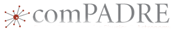# Performing Calculations using Measured Values that Include UncertaintyThis material was originally developed through comPADRE
as part of its collaboration with the SERC Pedagogic Service.

## Summary

In this activity, students practice performing calculations using measured values that include uncertainty. Students measure the mass (using an electronic balance) and volume (by water displacement) of pennies and use the values to calculate the density of the pennies. All measured values include uncertainty, and students practice using the rules for making calculations using numbers that include uncertainty.

As the students increase the number of pennies they use, the relative uncertainty of their calculated density decreases. Students will see factors that affect the uncertainty of each measurement and also how the uncertainty of each measurement contributes to the uncertainty of their calculated results.

Provided here is a data set for copper pennies (pre-1982) and zinc/copper pennies (post 1982). Students can use the data to identify what these pennies are made of, but only when the uncertainty of their calculated density is lower than the difference between the density of copper and the density of zinc.

## Learning Goals

This activity is a building block in the Measurement and Uncertainty module. This activity is intended to teach students to perform calculations using measurement values that include uncertainty. In addition, students will see an example of how to reduce the uncertainty of a measurement for volume by increasing the number of pennies they measure. Finally, they will practice making conclusions based on the amount of uncertainty in their results.

## Context for Use

This activity in intended for any science course where students are collecting and interpreting numerical data. Specifically, it is intended for use in course where teachers are using the methods described in the module Measurement and Uncertainty.

## Description and Teaching Materials

This activity can be done in the lab, as an interactive lecture demonstration, or as a worksheet. The worksheet provided here contains sufficient information to see how the lab would be conducted. worksheet for measuring the density of pennies (Microsoft Word 1MB Aug31 10)

If the activity is done in the lab, the worksheet provided here can be used as an assessment for the lab.

## Teaching Notes and Tips

Although the data (photos) provided in the worksheet above are sufficient to perform the calculations, it would be preferable for students to make the measurements themselves. No fancy equipment is needed -- just pennies, graduated cylinders, and some kind of scale or balance.

The composition of US pennies changed in 1981-82. Pre-1982 pennies are mostly copper and pennies made after 1982 are mostly zinc. Sort pennies into pre- and post- 1982 piles. Pennies made in 1982 could be either alloy, depending which mint made them. The US mint in Denver continued to make pennies of mostly copper through 1982. The instructor should sort the pennies into pre-1982, post-1982, and, for an extra mystery treat, 1982 D (Denver). This way, students will get different results and reach different conclusions, which makes for interesting class discussions.

## Assessment

If this activity is done using actual equipment, then the images included here can be used as a written assessment after the completion of the lab activity.

## References and Resources

Composition of the Cent from the US Mint - Includes a history of the composition of the US cent coin.